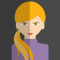## Find out the GCD of two numbers using while loop in C languageMandalika

Updated on 05-Mar-2021 11:17:21

ProblemGenerate the greatest common divisor for any two numbers using C programming language.SolutionLet the user enter any two numbers from the console. For those two numbers, let’s find the greatest common divisor.The GCD of two numbers is the largest number that exactly divides both of them without a remainder.The logic ... Read More

## Converting digits to word format using switch case in C languageMandalika

Updated on 05-Mar-2021 11:06:39

ProblemIs it possible to convert the given one or two-digit numbers into English words by using the C Programming language?SolutionWe can easily convert the given two-digit number into English word format with the help of a switch case. Not only two digits, but any number can also convert into English ... Read More

## Write a C program to find out the largest and smallest number in a seriesMandalika

Updated on 05-Mar-2021 11:00:04

ProblemLet the user enter four series of integers in the console, find out a number which is smallest and largest in a seriesSolutionTo calculate the small and large number, we use if conditions. The logic we use to find the largest and smallest number is −if(minno>q) //checking 1st and 2nd ... Read More

## Write a C program for time conversions using if and elseif statementsMandalika

Updated on 05-Mar-2021 10:54:51

ProblemHow to convert the time from 24 hr format to 12 hr format using C Programming language?SolutionRead the time value from the user (at run time). It has to be converted into 12 hr format from 24 hr.AlgorithmStart: Step 1: Enter time in 24 hr format Step 2: check the ... Read More

## How to print the stars in Diamond pattern using C language?Mandalika

Updated on 05-Mar-2021 10:48:51

Here, to print stars in diamond pattern, we are using nested for loops.The logic that we use to print stars in diamond pattern is shown below −//For upper half of the diamond the logic is: for (j = 1; j

## How to calculate transpose of a matrix using C program?Mandalika

Updated on 05-Mar-2021 10:44:53

Transpose of a matrixThe transpose of a matrix is the one whose rows are columns of the original matrix, i.e. if A and B are two matrices such that the rows of the matrix B are the columns of the matrix A then Matrix B is said to be the ... Read More

## Write a program to add two complex numbers using CMandalika

Updated on 05-Mar-2021 10:39:16

ProblemHow to add two complex numbers which are entered at run time by the user using C program −SolutionA complex number is a number that can be a combination of real and imaginary parts.It is represented in the form of a+ib.ProgramFor example, let’s take the two complex numbers as (4+2i) ... Read More

## How to print integers in the form of Pascal triangle using C?Mandalika

Updated on 05-Mar-2021 10:35:57

Pascal's triangle is the representation of integers in the form of a triangle. One of the famous representations of it is with binomial equations. We can use combinations and factorials to achieve this.Constructing a Pascal triangleAll values outside the triangle are considered zero (0). The first row is 0 1 ... Read More

## How to print Floyd’s triangle (of integers) using C program?Mandalika

Updated on 05-Mar-2021 10:31:09

Floyd's triangle is a right-angle triangle of consecutive numbers, starting with a 1 in the top left corner −For example,1 2 3 4 5 6 7 8 9 10Example 1 Live Demo#include int main(){    int rows, i,j, start = 1;    printf("Enter no of rows of Floyd's triangle :");    scanf("%d", &rows);    for (i = 1; i

## Check the number is Armstrong or not using CMandalika

Updated on 05-Mar-2021 10:27:39

ProblemHow to check whether the given number is an Armstrong number or not using C Programming language?SolutionArmstrong number is the number that is equal to the sum of cubes of its digits.Syntaxpqrs………=pow(p, n)+pow(q, n)+pow(r, n)+……….For example, 153, 371, 1634, etc., are Armstrong numbers.153=1*1*1 + 5*5*5 + 3*3*3    =1+125+27   ... Read More